##1. Cross Ratio

The cross ratio of four points {U,V,X,Y} on a conic (c) can be defined using a good parametrization on it. In fact, take an arbitrary line (e) and an additional point C on the conic and define (UVXY) to be equal to the cross ratio (U'V'X'Y'). By selecting another parametrization on the same or another line (e'), the corresponding "transition function" of the parametrizations is a homographic relation y=g(x) on the line coordinates of (e, e'). These relations preserve the cross ratios, thus (U'V'X'Y') = (U''V''X''Y'') and the definition is independent of the particular parametrization used. This means that it is independent of the line (e) and the location of point C on the conic.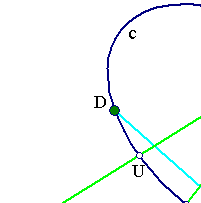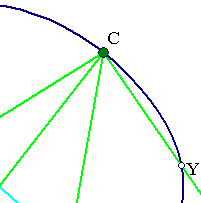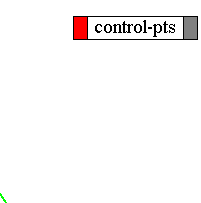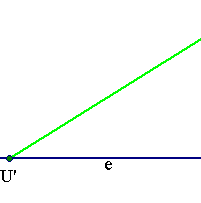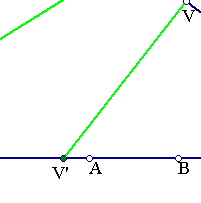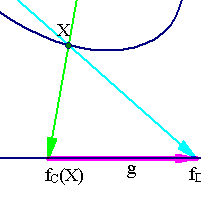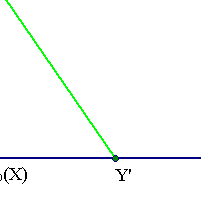##2. Haruki's Lemma

If AB and FD are nonintersecting chords in a circle and if P is a variable point on the arc AB remote from F and D, then for each position of P, the lines PF and PD cut AB into three segments of length x, y, z, where xz/y is constant.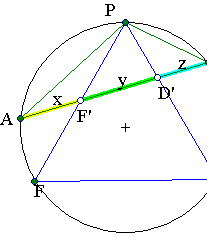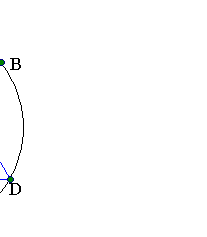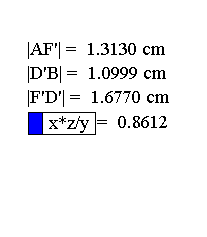Denote by d=|AB|. Then (x/y):(d/z) is the cross ratio of the four points {A,D,F,B} measured on line AB, and this is independent of the position of P. Obviously the property extends to chords of conics. The reduction of the property to the cross ratio of four points on a conic is due to Bankoff.

Chasles_Steiner.html
Chasles_Steiner_Envelope.html
Complex_Cross_Ratio.html
Complex_Cross_Ratio2.html
CrossRatio0.html
CrossRatioLines.html
FourTangentsCrossRatio.html GoodParametrization.html
Harmonic.html
Harmonic_Bundle.html